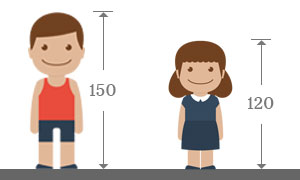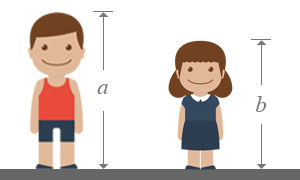# Unlike Algebraic Symbols

The algebraic symbols whose representing quantities are not same, are called unlike algebraic symbols.

Numbers are used to represent quantities in mathematics but symbols are used instead of the numbers to represent the quantities according to the algebraic mathematics. If a quantity is not equal to another quantity, they are called unlike quantities. If the algebraic symbols which represent those unlike quantities, they are known as unlike algebraic symbols algebraically.

## ExampleConsider heights of a boy and a girl.

It is measured that the height of a boy is $150$ centimetres and assume it is represented by a symbol $a$.

Therefore $a=150$

It is measured that the height of a girl is $120$ centimeters and assume it is denoted by a symbol $b$.

Therefore $b=120$Now, the height of the boy is written as $a$ instead of $150$ centimetres and the height of the girl is written as $b$ instead of $120$ centimetres.

The height of the boy is not equal to the height of the girl. So, the two quantities are known as unlike. Actually, the heights are now denoted by the letters $a$ and $b$. Therefore, $a$ is not equal to the $b$. In other words, $a\ne b$.

The alphabets $a$ and $b$ represents two different quantities. So, they are called as unlike algebraic symbols mathematically.

If two or more symbols represent different quantities, they are called as unlike algebraic symbols in mathematics.